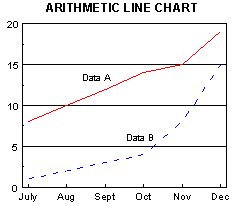Chart Pro online
User name (email):

 Recently used

 Forex

 Indices

# Charts

Because technical analysis is based on the study of data in the past, it is very important to present the data graphically. So charts are important element of technical analysis.

Data on the chart can be displayed in the so-called compressions. If there is no compression, this means that, for every trade separate point is drawn on chart. If the chart is in a specific compression, then each element of the chart corresponds to the value of financial instruments for the appropriate period. For example, daily chart (compression is one day) for each trading day has one element drawn. This rule is not applied to point and figure charts, you can see how they are constructed in section about point and figure charts how they are constructed.

Depending on the way how elements of the chart are drawn, there are different types of charts:

Chart can be drawn in arithmetic or logarithmic scale. For arithmetic charts vertical axis has equidistant distances. For example, distance between 1 and 2 is same as between 2 and 3, as well as between 3 and 4 and so on. On a logarithmic chart the distances are different. Distances are smaller as price increases. The advantage of logarithmic scale is that equal percentage changes have the same distance. Therefore price change from 10 to 20 (100%) is the same as the change from 20 to 40, or from 40 to 80 etc. Logarithmic charts point out the percentage change, but their construction is a bit complicated and they can not show negative values (which is required to view certain indicators). Therefore, in most cases users are using the arithmetic charts, while the logarithmic charts are more interesting for advance users.